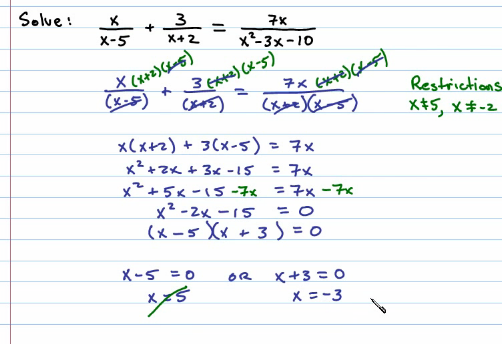Friday, December 2, 2011

Solving Rational Equations in Beginning Algebra

A thorough explanation for solving rational equations can be read for free in the Elementary Algebra textbook that is available on the Flat World Knowledge website:

Also, you can click on the following rational equation to watch a video solution.

The basic steps for solving these equations are as follows:

Step 1: Factor all denominators and determine the LCD.
Step 2: Identify the restrictions.
Step 3: Multiply both sides of the equation by the LCD. Distribute carefully and then simplify.
Step 4: Solve the resulting equation.
Step 5: Check for extraneous solutions.

The complete solution is presented below:Basically, we multiply both sides of the equation by the LCD as a means to clear the fractions.  This introduces the possibility for extraneous solutions and so we must check our answers.  Look for more Chapter 7 videos here.